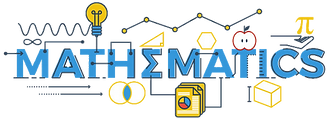Add domino dots. Children count the dots on each half of the domino. Add them together then drag the correct number over the total number of dots on each domino.

Addition circles (1). A simple 3 stage addition activity designed for PreK and special needs students and situations where teachers may be working one on one with children.
Addition circles (2). As (1) Numbers 1 through 5.
Addition circles (3). As (1) Numbers 1 through 9.

Bear bonds to 10. Number bonds to ten.  Children drop numbers on to the bears' paws to total ten.
Bear bonds to 20.  Number bonds to 20.  Children drop numbers on to the bowling balls to total 20.

Count domino dots.  Children work together counting domino dots and matching them with their correct number. Positions of all objects change every time the activity runs.

Count pennies to 5 (1). The aim of the activity is to help children to count to 5 using pennies.  Drag the pennies on to the pre-defined places on the surface.
Count pennies to 5 (2).   The pennies are not in numeric sequence this time, and the order will change each time the activity runs.

Counting farm animals. Counting 1 to 9. Counting practice and recognizing grouping patterns.

Counting in 10s to 100. Children sequence the dominoes matching the words with the numbers and increasing by 10. Objectives:  Children will practice sequencing numerals 0-100 by tens. Children will be able to skip count orally by 10's to 100.

Finger counting (1).  This activity is designed to help students recognize numbers and relate them to counting on their fingers. Number cards should be matched with finger cards.
Finger counting (2).  Finger cards should be matched with number cards.

Finger sums threes.  This simple finger sums activity has additions to 10 using three's.
Finger sums twos.    This simple finger sums activity has additions to 10 using two's.

Make sequence activity.  Order five piles of coins from smallest to largest.

Multiply to 12 dominoes.  Domino loops to practice multiplication up to x12. Children could also divide up the dominoes and play "I have, who has"?

Sequencing coin values. Sequence the coins from left to right to show increasing value.

Subtraction circles.  A simple 3 stage subtraction activity designed for PreK and special needs students and situations where teachers may be working one on one with children. Numbers 1 through 9.

Subtraction leaves from 5.   A very simple subtraction activity (using real numbers) designed for early learners or special needs students.  Ideally an adult and a child would be working in a one on one situation.

Washing line to 10.  Children recognize numbers 1 through 10 and sequence them in correct order from left to right on the washing lines.

## Activity House App